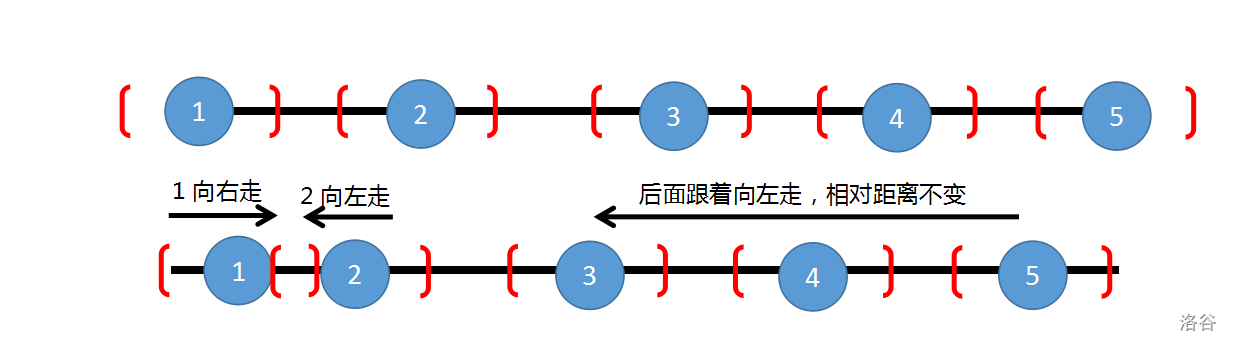# Doubeecat's Blog

“即便前路混沌，同她走过，才算人间。”

0%

P6397 [COI2008] GLASNICI

• 在任意时刻（不一定是整数时刻），任一信使（无论是否已知消息）都可以自由选择向左移动或者向右移动或者原地不动。其移动的速度为每秒 $1$ 单位长度。
• 当两个信使相距不超过一给定实数 $k$ 时，双方可以进行消息传递，也即如果两人中有一人已知该消息，则两人都知道了该消息。消息传递是瞬间发生的，不消耗时间。

$1 \leq n \leq 10^5,0 \leq k \leq 10^6$

## 解题思路1. $now + k \geq x_{i+1}$ 这种情况就贪心的让 $now = now + k$
2. $now + k < x_{i+1}$ 这种情况我们没有办法，只能让 $now = x_i + k$
3. $now < x_i - k - mid$ 这样无论怎么都到达不了了， $mid$ 就肯定不能取到。